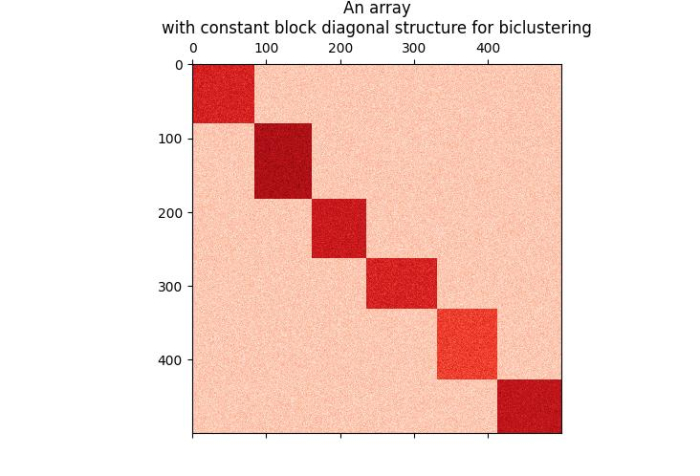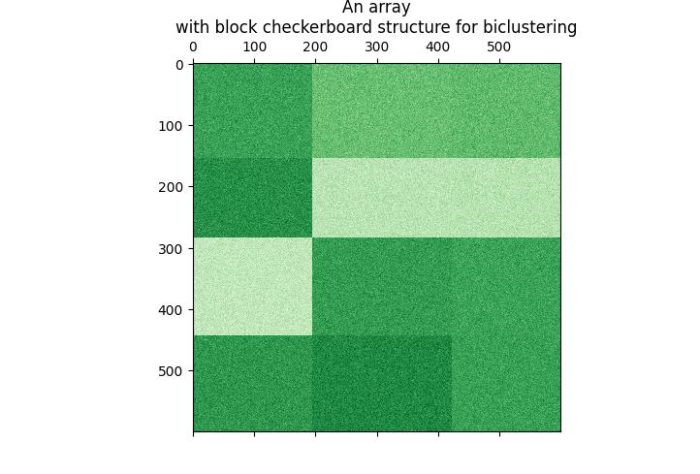# How to generate an array for bi-clustering using Scikit-learn?

In this tutorial, we will learn how to generate an array with a constant block diagonal structure and with a block checkerboard structure for bi-clustering using Python Scikit-learn (Sklearn).

## Generating an Array with a Constant Block Diagonal Structure

To generate an array with constant block diagonal structure for biclustering, we can take the following steps −

Step 1 − Import sklearn.datasets.make_biclusters and matplotlib.

Step 2 − Set the figure size

Step 3 − Create data points namely data, row, and column.

Step 4 − Create a plotter to show the array with constant block diagonal structure.

Step 5 − Provide title.

### Example

In the below given example, we will be generating an array of shape (500, 500) with 6 clusters.

# Importing libraries
from sklearn.datasets import make_biclusters

# Matplotlib for plotting the array with constant diagonal structure
from matplotlib import pyplot as plt

# Set the figure size
plt.rcParams["figure.figsize"] = [7.50, 3.50]
plt.rcParams["figure.autolayout"] = True

# Creating the bi-cluster Test Datasets using sklearn.datasets.make_biclusters

data, rows, columns = make_biclusters(
shape=(500, 500), n_clusters=6, noise=5, shuffle=False, random_state=0
)
plt.matshow(data, cmap=plt.cm.Reds)

plt.title("An array\nwith constant block diagonal structure for biclustering")

plt.show()


### Output

It will produce the following output −## Generating an Array with Block Checkerboard Structure

To generate an array with block checkerboard structure for biclustering, we can take the following steps −

Step 1 − Import the libraries sklearn.datasets.make_checkerboard and matplotlib.

Step 2 − Set the figure size

Step 3 − Create data points namely data, row, and column

Step 4 − Create a plotter to show the array with constant block diagonal structure.

Step 5 − Provide title.

### Example

In the below given example, we will be generating an array of shape (600, 600) with number of clusters = (4, 3).

# Importing libraries
from sklearn.datasets import make_checkerboard

# Matplotlib for plotting the array with block chekerboard structure
from matplotlib import pyplot as plt

# Set the figure size
plt.rcParams["figure.figsize"] = [7.50, 3.50]
plt.rcParams["figure.autolayout"] = True

# Creating the Test Datasets using sklearn.datasets.make_checkerboard

n_clusters = (4, 3)
data, rows, columns = make_checkerboard(
shape=(600, 600), n_clusters=n_clusters, noise=10, shuffle=False, random_state=0
)
plt.matshow(data, cmap=plt.cm.Greens)

plt.title("An array\nwith block checkerboard structure for biclustering")

plt.show()


### Output

It will produce the following output −